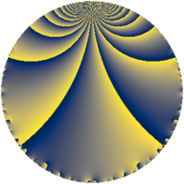# Properties

 Label 39.2.jLevel $39$ Weight $2$ Character orbit 39.j Rep. character $\chi_{39}(4,\cdot)$ Character field $\Q(\zeta_{6})$ Dimension $2$ Newform subspaces $1$ Sturm bound $9$ Trace bound $0$

# Related objects

## Defining parameters

 Level: $$N$$ $$=$$ $$39 = 3 \cdot 13$$ Weight: $$k$$ $$=$$ $$2$$ Character orbit: $$[\chi]$$ $$=$$ 39.j (of order $$6$$ and degree $$2$$) Character conductor: $$\operatorname{cond}(\chi)$$ $$=$$ $$13$$ Character field: $$\Q(\zeta_{6})$$ Newform subspaces: $$1$$ Sturm bound: $$9$$ Trace bound: $$0$$

## Dimensions

The following table gives the dimensions of various subspaces of $$M_{2}(39, [\chi])$$.

Total New Old
Modular forms 14 2 12
Cusp forms 6 2 4
Eisenstein series 8 0 8

## Trace form

 $$2 q + q^{3} - 2 q^{4} - 3 q^{7} - q^{9} + O(q^{10})$$ $$2 q + q^{3} - 2 q^{4} - 3 q^{7} - q^{9} - 6 q^{11} - 4 q^{12} + 7 q^{13} + 6 q^{15} - 4 q^{16} + 6 q^{19} + 12 q^{20} + 6 q^{23} - 14 q^{25} - 2 q^{27} + 6 q^{28} - 6 q^{29} - 6 q^{33} - 6 q^{35} - 2 q^{36} + 2 q^{39} - 12 q^{41} + q^{43} + 6 q^{45} + 4 q^{48} - 4 q^{49} - 10 q^{52} + 24 q^{53} + 12 q^{55} - 6 q^{59} - q^{61} + 3 q^{63} + 16 q^{64} + 6 q^{65} + 15 q^{67} - 6 q^{69} - 18 q^{71} - 7 q^{75} - 12 q^{76} + 12 q^{77} - 22 q^{79} - 24 q^{80} - q^{81} + 6 q^{84} + 6 q^{87} + 12 q^{89} - 9 q^{91} - 24 q^{92} + 3 q^{93} + 12 q^{95} - 9 q^{97} + O(q^{100})$$

## Decomposition of $$S_{2}^{\mathrm{new}}(39, [\chi])$$ into newform subspaces

Label Dim $A$ Field CM Traces $q$-expansion
$a_{2}$ $a_{3}$ $a_{5}$ $a_{7}$
39.2.j.a $2$ $0.311$ $$\Q(\sqrt{-3})$$ None $$0$$ $$1$$ $$0$$ $$-3$$ $$q+(1-\zeta_{6})q^{3}-2\zeta_{6}q^{4}+(-2+4\zeta_{6})q^{5}+\cdots$$

## Decomposition of $$S_{2}^{\mathrm{old}}(39, [\chi])$$ into lower level spaces

$$S_{2}^{\mathrm{old}}(39, [\chi]) \cong$$ $$S_{2}^{\mathrm{new}}(13, [\chi])$$$$^{\oplus 2}$$